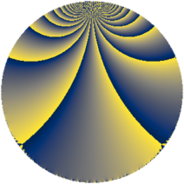# Properties

 Label 672.4.bqLevel $672$ Weight $4$ Character orbit 672.bq Rep. character $\chi_{672}(85,\cdot)$ Character field $\Q(\zeta_{8})$ Dimension $576$ Sturm bound $512$

# Related objects

## Defining parameters

 Level: $$N$$ $$=$$ $$672 = 2^{5} \cdot 3 \cdot 7$$ Weight: $$k$$ $$=$$ $$4$$ Character orbit: $$[\chi]$$ $$=$$ 672.bq (of order $$8$$ and degree $$4$$) Character conductor: $$\operatorname{cond}(\chi)$$ $$=$$ $$32$$ Character field: $$\Q(\zeta_{8})$$ Sturm bound: $$512$$

## Dimensions

The following table gives the dimensions of various subspaces of $$M_{4}(672, [\chi])$$.

Total New Old
Modular forms 1552 576 976
Cusp forms 1520 576 944
Eisenstein series 32 0 32

## Trace form

 $$576q + O(q^{10})$$ $$576q + 240q^{10} - 96q^{12} - 120q^{16} - 72q^{18} + 392q^{22} + 656q^{23} + 912q^{24} + 80q^{26} + 2480q^{32} + 2000q^{34} - 3280q^{40} + 1616q^{43} - 1000q^{44} - 2880q^{46} - 2976q^{51} - 1728q^{52} + 1504q^{53} - 432q^{54} - 576q^{55} + 392q^{56} + 2448q^{60} - 3648q^{61} + 5856q^{62} + 1008q^{63} + 816q^{67} + 10064q^{68} - 2112q^{69} + 1008q^{70} - 2632q^{74} - 4416q^{75} - 10064q^{76} + 3808q^{77} - 12640q^{80} - 11680q^{82} - 4736q^{86} + 3120q^{88} + 2152q^{92} + 23600q^{94} + O(q^{100})$$

## Decomposition of $$S_{4}^{\mathrm{new}}(672, [\chi])$$ into newform subspaces

The newforms in this space have not yet been added to the LMFDB.

## Decomposition of $$S_{4}^{\mathrm{old}}(672, [\chi])$$ into lower level spaces

$$S_{4}^{\mathrm{old}}(672, [\chi]) \cong$$ $$S_{4}^{\mathrm{new}}(32, [\chi])$$$$^{\oplus 4}$$$$\oplus$$$$S_{4}^{\mathrm{new}}(96, [\chi])$$$$^{\oplus 2}$$$$\oplus$$$$S_{4}^{\mathrm{new}}(224, [\chi])$$$$^{\oplus 2}$$